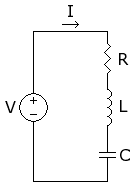# Voltage across R,L,C

I am trying to find the effective voltage across each element (R,L,C) of a series RLC circuit like the one below. I am given the following values: Ieff = 1 Amp, Veff = 1 V, i(t) lags v(t) by 45°, L = 1, f = 2/2∏ Hz, L = 1 HWhat I have done so far:
Veff = Vm/√2
=> Vm = √2
Ieff = Im/√2
=> Im = √2
XL = ωL = 2

v(t) = √2*sin(2t)
i(t) = √2*sin(2t - 45°)

i know the formulas for the peak voltages for the individual elements are
VR = Im*R
VL = Im*XL
VC = Im*XC

i'm not sure if those correspond to the effective voltages or if i have to use the equation Veff = Vm/√2
i am also having trouble finding R and XC from the information provided..

Last edited:

I agree with your value for Xl.
If the current lags the supply voltage then the supply voltage LEADS the voltage across R because the voltage across R and the current are in phase.
Are you familiar with showing the voltages Vl, Vr, and Vc on a vector (phasor) diagram. Vl is drawn on the =+y axis, Vr is drawn on the +X axis (horizontal axis ) and Vc is drawn on the -y axis.
This means, from the information in your question, that the supply voltage (1volt) will be a vector from the origin at 45 degrees to the X axis.
Hope this is of some help
I think that you have not been given enough information! Do you know anything about R or C?

Sorry....you do have enough information,I had missed the current

Imax = Vmax/Z
=> Z = 1
(1) Z = sqrt(R2 + (XL - XC)2)
cosθ = R/Z, θ = 45°
1/√2 = R/1
R = 1/√2
From (1) I get C = 1/(4-√2)

I am not 100% sure this is correct

I got those values for R and C

I got those values for R and C

thanks for the help. i got
VR = Im*R = 1
VL = Im*XL = 2√2
VC = Im*XC = 2√2 - 1

I got
Vr = 1 x Cos45 =0.7V
R = 0.7/1 = 0.7Ω
Vc = 1.3V
Xc = 1.3 (gives C= 0.38F)

I think the differences are due to the fact that I used the voltage and current values in the question. I assume these are rms values and I think you have converted these to peak values.

A good result !!
Cheers